# Section 3 Reactions in Aqueous Solutions Terms to

• Slides: 8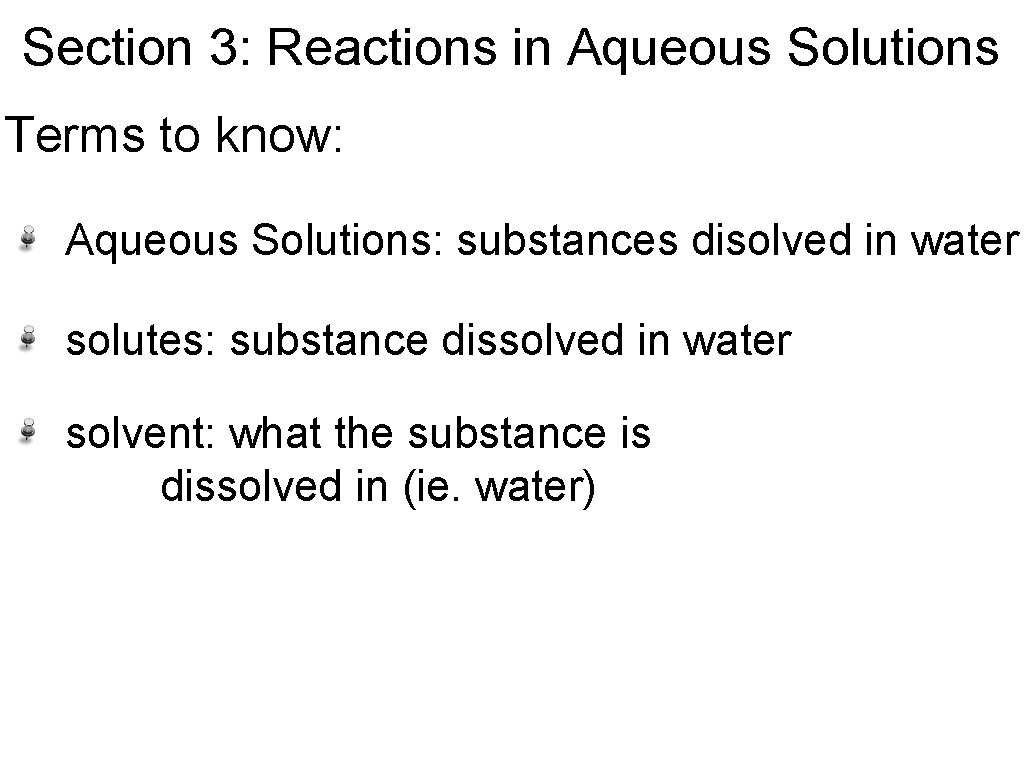Section 3: Reactions in Aqueous Solutions Terms to know: Aqueous Solutions: substances disolved in water solutes: substance dissolved in water solvent: what the substance is dissolved in (ie. water)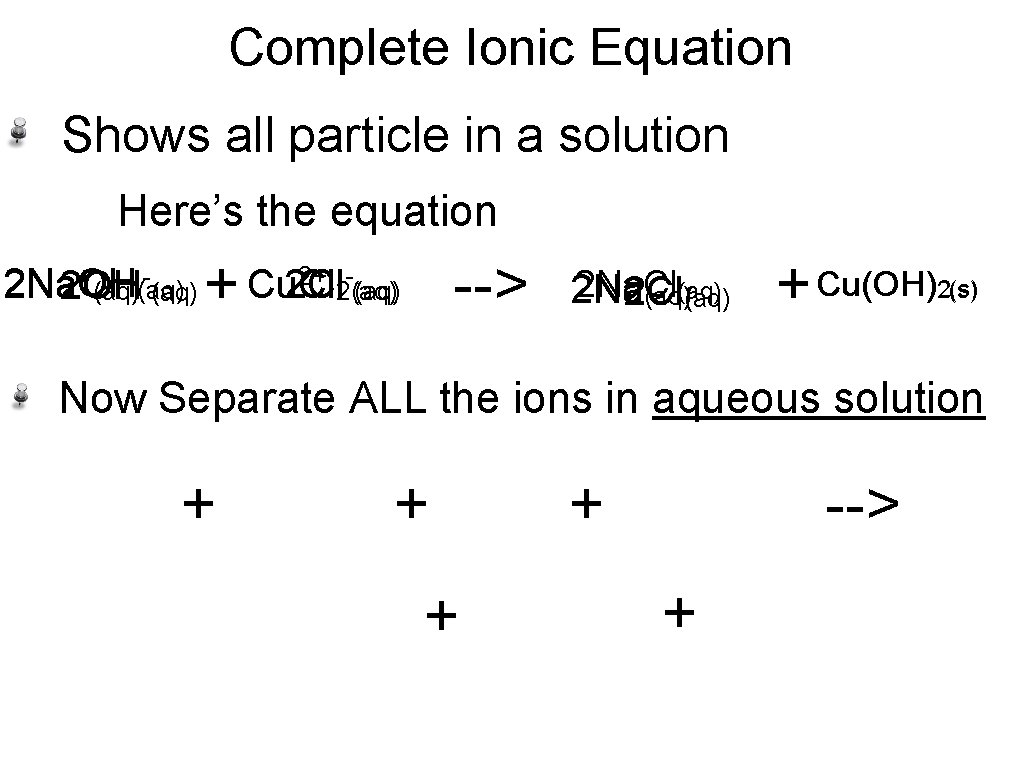Complete Ionic Equation Shows all particle in a solution Here’s the equation + 2+ 2 Na. OH 2 Na Cu. Cl Cu 2 Cl 2 OH (aq) 2(aq) + --> 2 Na. Cl (aq) 2 Na 2 Cl (aq) + Cu(OH) 2(s) Now Separate ALL the ions in aqueous solution + + --> +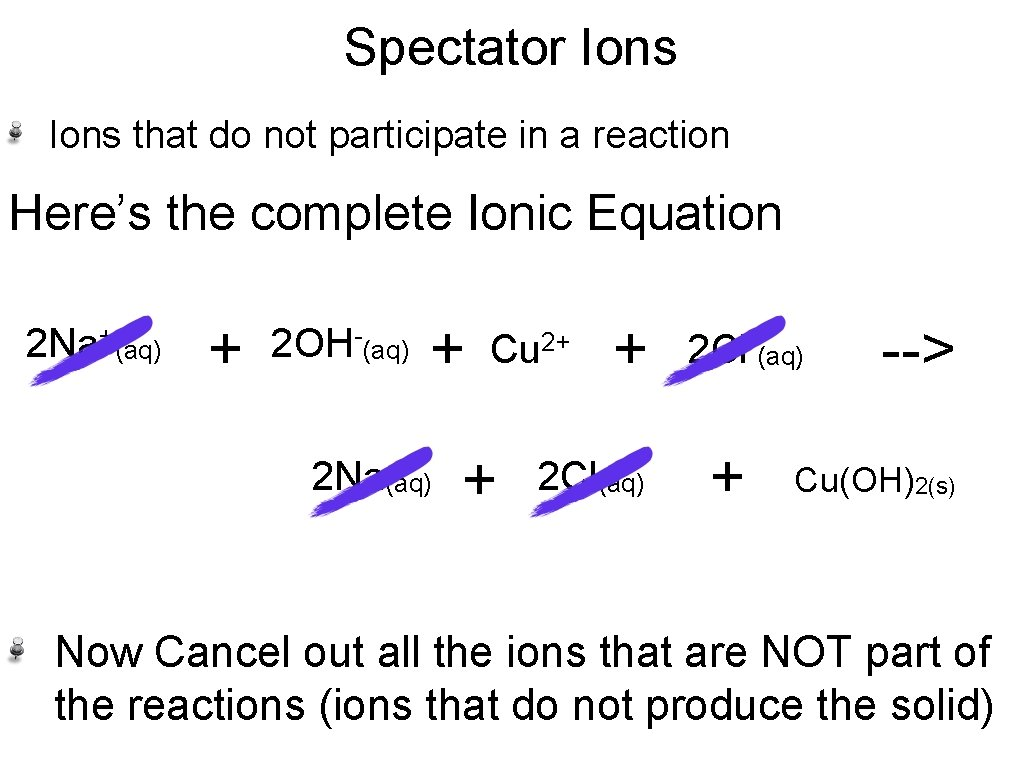Spectator Ions that do not participate in a reaction Here’s the complete Ionic Equation 2 Na+(aq) + 2 OH-(aq) + 2 Na(aq) Cu 2+ + + 2 Cl(aq) 2 Cl-(aq) + --> Cu(OH)2(s) Now Cancel out all the ions that are NOT part of the reactions (ions that do not produce the solid)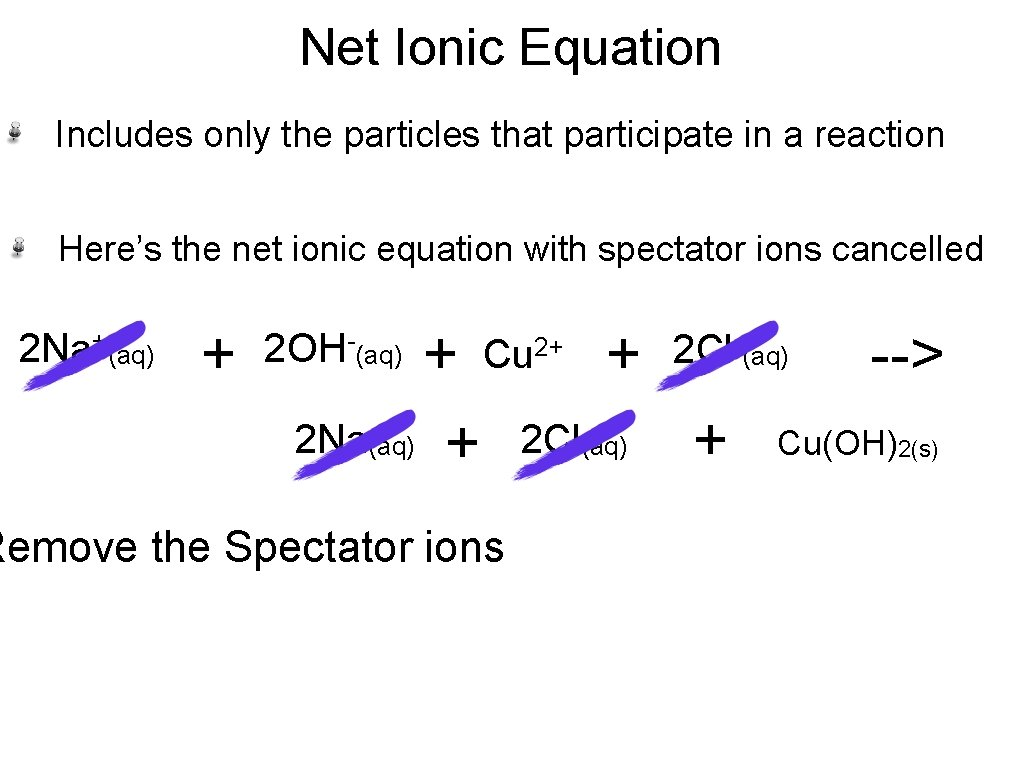Net Ionic Equation Includes only the particles that participate in a reaction Here’s the net ionic equation with spectator ions cancelled 2 Na+(aq) + + 2 Na(aq) + 2 OH-(aq) 2+ Cu Remove the Spectator ions + 2 Cl(aq) 2 Cl-(aq) + --> Cu(OH)2(s)3 Types of Aqueous Reactions 1. Reactions that form Precipitates (solids) 2 Na. OH(aq) + Cu. Cl 2(aq) --> 2 Na. Cl(aq) + Cu(OH)2(s). 2. Reactions that form Water HBr(aq) + Na. OH(aq) --> H 2 O(l) + Na. Br(aq) 3. Reactions that form Gases 2 HI(aq) + Li 2 S(aq) --> H 2 S(g) + 2 Li. I(aq)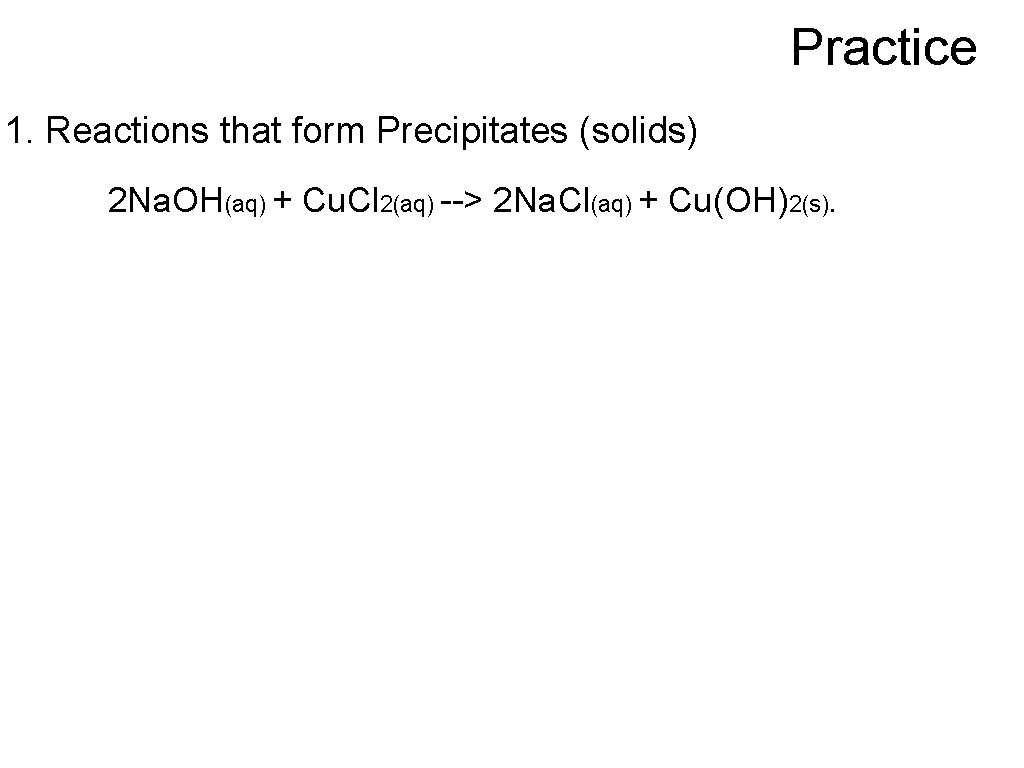Practice 1. Reactions that form Precipitates (solids) 2 Na. OH(aq) + Cu. Cl 2(aq) --> 2 Na. Cl(aq) + Cu(OH)2(s).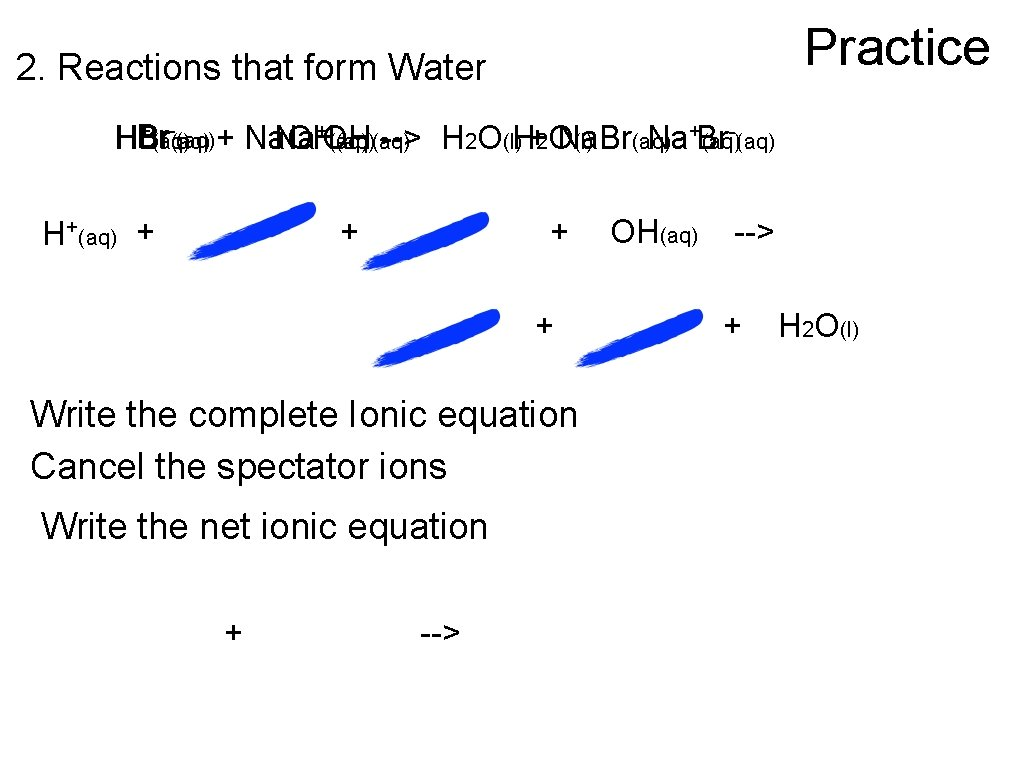Practice 2. Reactions that form Water -(aq) +(aq) -(aq) + Na. OH HBr Na+OH (aq)(aq) --> H 2 O(l)H+2 O Na. Br (l) (aq) Na+Br (aq) H+(aq) + + Write the complete Ionic equation Cancel the spectator ions Write the net ionic equation + --> OH(aq) --> + H 2 O(l)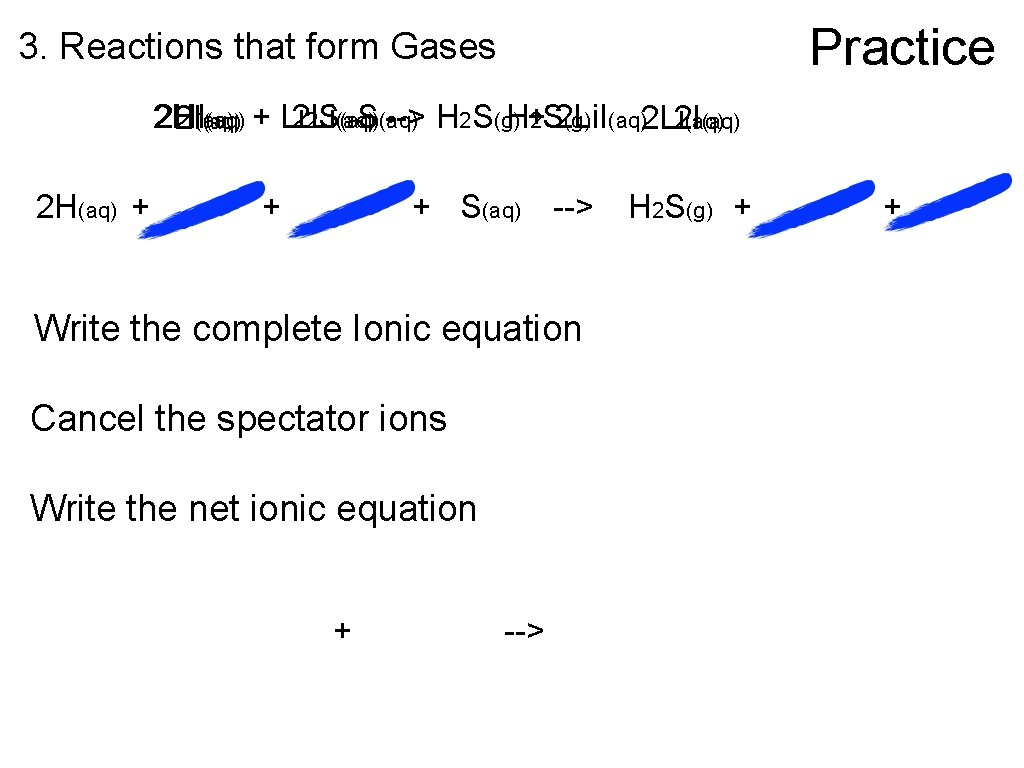Practice 3. Reactions that form Gases 2 H 2 HI (aq) + Li 2 S(aq) --> H 2 S(g)H+2 S 2 Li. I (g) (aq)2 Li 2 I(aq) 2 H(aq) + + + S(aq) --> Write the complete Ionic equation Cancel the spectator ions Write the net ionic equation + --> H 2 S(g) + +